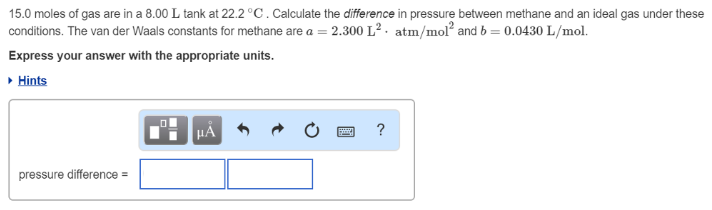# Problem: 15.0 moles of gas are in a 8.00 L tank at 22.2°C. Calculate the difference in pressure between methane and an ideal gas under these conditions. The van der Waals constants for methane are a = 2.300 L2 • atm/mol2 and b = 0.0430 L/mol. Express your answer with the appropriate units.

###### FREE Expert Solution
95% (484 ratings)###### Problem Details

15.0 moles of gas are in a 8.00 L tank at 22.2°C. Calculate the difference in pressure between methane and an ideal gas under these conditions. The van der Waals constants for methane are a = 2.300 L2 • atm/mol2 and b = 0.0430 L/mol.